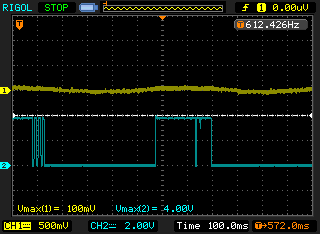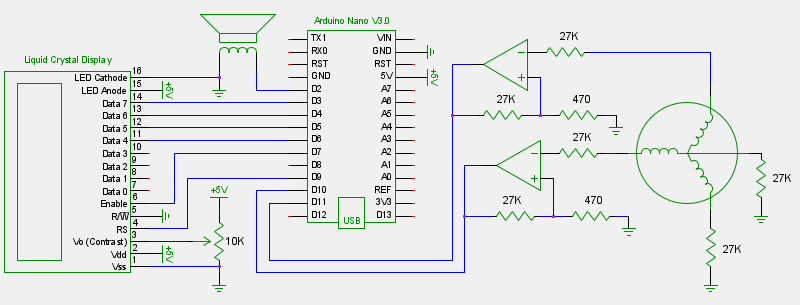## Brushless Motors as Rotary Encoders

#### May 20, 2012

Brushless motors can be used as rotary encoders. When the rotor is turned the three coils will produce AC waveforms, each one-third out of phase with the next coil. The period and amplitude depend on how fast the rotor is turned. Spinning the rotor faster will result in shorter periods and higher amplitudes. Feeding those waveforms into op amps does the trick. For this test I displayed a number on an LCD and used a buzzer to play a 5KHz tone for each clockwise movement and a 1KHz tone for each counter-clockwise movement. The motor is from an old floppy disk drive.The op amps are used to turn each AC waveform into a square wave that can be interpreted by the microcontroller:If an op amp is used as a simple comparator (waveform going to one input, with the other input tied to ground) there will be problems if you spin the rotor slowly or very fast. The slightest amount of noise will cause glitches. (The output will swing high or low when it shouldn't.)A little positive feedback adds hysteresis to the circuit. This is simply a threshold which must be crossed in order to get the output to swing high or low. For my particular motor I needed the threshold to be approximately 70mV. If the threshold is too low noise can still get through and cause problems. If the threshold is too high you will not be able to sense slow rotations. The sweet spot will be different for every motor.

Positive feedback supplies part of the op amp output back into the non-inverting input. A voltage divider is used to provide the feedback, one end goes to the op amp output and the other end goes to ground. To get 70mV when the output is high I needed two resistors with a 54.3:1 ratio. To find the x:1 ratio, use: x = (output high voltage) / (desired mV) * 1000. To minimize current flow the two resistors should add up to more than 1K. The op amps I used will output about 3.8V when supplied with 5V so I used some 27K and 470 resistors that I had laying around which gave a 66mV threshold.

When an op amp input has a voltage below the negative supply voltage, most op amps will effectively short that input to ground through a diode. Since the motor is only being spun by hand this will not be a problem but some current limiting resistors should be in series with all motor leads to be on the safe side. Also keep the op amp voltage limits in mind when using larger motors or if you will be spinning the rotor at a significant speed.

I initially set up three op amps, one for each coil. This resulted in noticeable glitching at low speeds. The resistors I used for positive feedback have a 5% tolerance, resulting in the threshold for each coil being slightly different. I'm fairly certain that was the cause of the problem but I may be wrong. There is still a little glitching when using just two coils but it occurs far less often.

Here's the final schematic and code:```#include <LiquidCrystal.h> LiquidCrystal lcd(9,7,6,5,4,3); // RS, Enable, D4, D5, D6, D7 int count = 0; byte currentA, currentB, previousA, previousB = 0; void setup() { lcd.begin(16, 2); previousA = currentA = digitalRead(10); previousB = currentB = digitalRead(11); } void loop() { previousA = currentA; previousB = currentB; currentA = digitalRead(10); currentB = digitalRead(11); if(previousA == LOW && previousB == LOW && currentA == HIGH && currentB == LOW) { // clockwise count++; tone(2, 5000, 2); } else if(previousA == HIGH && previousB == HIGH && currentA == LOW && currentB == HIGH) { // clockwise count++; tone(2, 5000, 2); } else if(previousA == LOW && previousB == LOW && currentA == LOW && currentB == HIGH) { // counter-clockwise count--; tone(2, 1000, 2); } else if(previousA == HIGH && previousB == HIGH && currentA == HIGH && currentB == LOW) { // counter-clockwise count--; tone(2, 1000, 2); } lcd.setCursor(0, 0); lcd.print(count); lcd.print(" "); }```